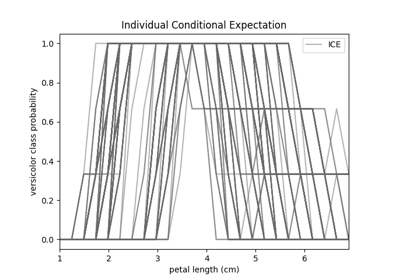# fatf.vis.feature_influence.plot_individual_conditional_expectation¶

fatf.vis.feature_influence.plot_individual_conditional_expectation(ice_array: numpy.ndarray, feature_linespace: numpy.ndarray, class_index: int, feature_name: Optional[str] = None, class_name: Optional[str] = None, plot_axis: Optional[matplotlib.axes._axes.Axes] = None) → Tuple[Optional[matplotlib.figure.Figure], matplotlib.axes._axes.Axes][source]

Plots Individual Conditional Expectation for a selected class.

Parameters
ice_arraynumpy.ndarray

An array of (n_samples, n_steps, n_classes) shape with Individual Conditional Expectation calculation results for every target class for the desired spectrum of the selected feature. This should be the output of the fatf.transparency.models.feature_influence.individual_conditional_expectation function.

feature_linespacenumpy.ndarray

A one-dimensional array – (steps_number, ) – with the values for which the selected feature was sampled when the dataset was evaluated for a predictive model. This should be the output of the fatf.transparency.models.feature_influence.individual_conditional_expectation function.

class_indexinteger

The index of the class for which ICE will be plotted, taken from the original dataset.

feature_namestring, optional (default=None)

The name of the feature for which ICE was originally calculated.

class_namestring, optional (default=None)

The name of the class that class_index parameter points to. If None, the class name will be the same as the class index.

plot_axismatplotlib.pyplot.Axes, optional (default=None)

A matplotlib axes on which the ICE will be plotted. This is useful if one wants to overlay multiple ICE plot on top of each other. If None, a new axes will be created.

Returns
plot_figureUnion[matplotlib.pyplot.Figure, None]

A matplotlib figure that holds the plot_axis axis. This parameter is None when the user passed in plot_axis attribute, otherwise, when a blank plot is created, this is a figure object holding the plot axis (plot_axis).

plot_axismatplotlib.pyplot.Axes

A matplotlib axes with the ICE plot.

Raises
IncorrectShapeError

The ICE or the PD array has a wrong number of dimensions (3 and 2 respectively). The feature linespace array has a wrong number of dimensions – 1 is expected.

IndexError

The class index is invalid for the input array.

TypeError

The class index is not an integer; the feature name is not a string or a None; the class name is not a string or a None; the plot axis is not a matplotlib.pyplot.Axes type object or a None.

ValueError

The input array is structured or is not numerical. The linespace array is structured, not numerical or its length does not agree with the number of steps in the input array. If the plot_axis attribute is provided, and is not None this exception will be raised if the range of either of the axes does not agree with the range of the axes of the plot_axis plot.

## Examples using fatf.vis.feature_influence.plot_individual_conditional_expectation¶